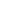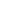Secrets Of Quran Miracles

Site Of Abduldaem Al-Kaheel

•••New Numeric MiracleWe can say: In every number, there is a wonderful miracle, because the Quran is the book of Allah…

In the name of Allah, The Most Gracious The Most Merciful, Allah says:

"Let them then produce a recital like unto it if (it be) they speak the Truth!" (The Tur chapter :34)

The numbers that Allah has chosen for His holy book (The Koran) no human kind can ever produce like them. Allah, Glorified be Him, has chosen for his book:

• The number of verses: 6236 verses
• The number of chapters: 114 chapters
• The number of the years of revelation: 23 years.

Then these special numbers found in the Qur’an fit a very big number of equations that would never be realized by other numbers…and the following are some of these equations; the numbers are ranged from big to small.

The number of the koranic chapters 114: its characteristic is that the number of the first chapter and that of the last one constitute the number of the number 114, and it is a multiple of the number 7; moreover, the total of its numbers gives the sum of 7: ( 1+1+4+1=7)

The years of revelation 23: it has the same feature, in the sense that the first year and the last one constitute the number of 231 ( a multiple of 7).

The verses and the chapters constitute the number of 1146236 which is about seven categories and can be divided by 7 in the two senses; the sum of its numbers too gives 23 ( the number of the years of revelation):

1+1+4+6+2+3+6=23

The total of the singles of the three numbers:

2+3+1+1+4+6+2+3+6= 28=7×4

The number 28 has a very important significance, i.e. it represents the number of the letters of the Arabic ABC (the language of the Koran)

The chapter 114 and the years 23: the constitute a number that is a multiple of 7: 23114, and the total of the numbers of each is:

2+3=5

1+1+4=6

then 56 is a multiple of 7.

The verses and the years: they constitute a number that is multiple of 7 was too: 236236

The verse which talks about the divine protection of the holy Koran:

"We have without doubt sent down the Message; and We will assuredly guard it (from corruption" (Al Hijr chapter: 9).

The number of the letters in this verse is 28, the same number of the letters of the Arabic ABC!!!

Moreover, the number of this verse (which talks about the protection of the Koran) wonderfully agrees with the number of the Koranic chapters as following:

The verses together with the number of this verse gives 96236 which is a multiple of 7 for two times.

The chapters together with the number of this verse gives 9114 which is also a multiple of 7 for two times.

To conclude, nothing is left but to mention that each of these three numbers  has a limited number of classes:

23114 is a number of 5 classes

236236 is a number of 6 classes

1146236 is a number of 7 classes

and if we range the three numbers, we will have 567 (a multiple of 7).

So look how wonderful and miraculous these agreements between 7 and the three numbers (the number of verses, of chapters and of the years of revelation), and this is only part of the many equations. Then, researches that have been published contain more equations and more agreements between 7 and other prime numbers which have a koranic significance.

Therefore, we have to ask a question to those who have claimed they can produce such divine book:

‘Can you bring these three numbers 23-114-6236 only? so if you can not do, just remember Allah’s saying:

"And if you are in doubt as to what We have revealed from time to time to Our servant then produce a Surah like thereunto; and call your witnesses or helpers (if there are any) besides Allah if your (doubts) are true. But if you cannot and of a surety you cannot then fear the fire whose fuel is Men and Stones which is prepared for those who reject Faith. (The Cow chapter: 23-24)

-------------------

By: Abduldaem Al-Kaheel

Sources:

- The Encyclopaedia of the Numeric Miracles in the Holy Quran. By: Abduldaem Al-Kaheel.Secrets Of Quran Miracles – Site Of Abduldaem Al-Kaheel

All articles in this site are free